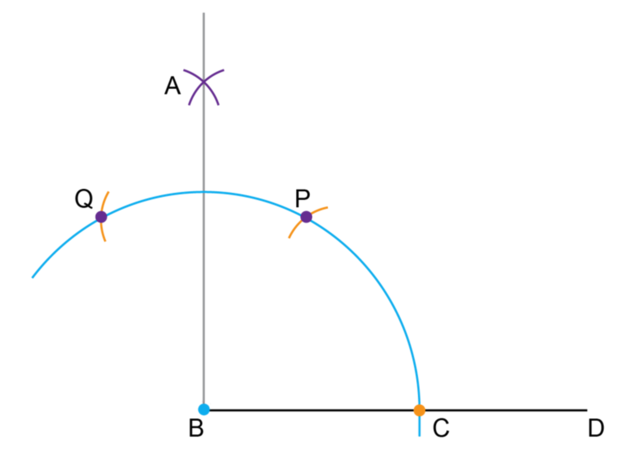Home Practice
For learners and parents For teachers and schools
Textbooks
Full catalogue
Pricing SupportLog in

We think you are located in United States. Is this correct?

# Constructing special angles

## 10.5 Constructing special angles

In this section, you will learn how to construct $$30^{\circ}$$, $$45^{\circ}$$ and $$60^{\circ}$$ angles using a compass. You will also learn how to construct multiples or combinations of these angles.

### Complementary and supplementary angles

When the sum of two angles is equal to $$90^{\circ}$$, we say that the two angles are complementary angles.

For example:

• $$\hat{A} = 50^{\circ}$$, $$\hat{B} = 40^{\circ}$$
• $$50^{\circ} + 40^{\circ} = 90^{\circ}$$
• $$\hat{A}$$ and $$\hat{B}$$ are complementary angles.
• $$\hat{A}$$ is the complement of $$\hat{B}$$.
• $$\hat{B}$$ is the complement of $$\hat{A}$$.
complementary angles
two angles that have a sum of $$90^{\circ}$$

When the sum of two angles is equal to $$180^{\circ}$$, we say that the two angles are supplementary angles.

For example:

• $$\hat{C} = 110^{\circ}$$, $$\hat{D} = 70^{\circ}$$
• $$110^{\circ} + 70^{\circ} = 180^{\circ}$$
• $$\hat{C}$$ and $$\hat{D}$$ are supplementary angles.
• $$\hat{C}$$ is the supplement of $$\hat{D}$$.
• $$\hat{D}$$ is the supplement of $$\hat{C}$$.
supplementary angles
two angles that have a sum of $$180^{\circ}$$

## Worked Example 10.5: Calculating complementary angles

$$\hat{T}$$ and $$\hat{S}$$ are complementary angles. If $$\hat{S} = 37^{\circ}$$, determine $$\hat{T}$$.

### Recall the definition of complementary angles.

We know that complementary angles are two angles that together have a sum of $$90^{\circ}$$.

So:

\begin{align} \hat{T} + \hat{S} &= 90^{\circ} \\ \hat{T} &= 90^{\circ} − \hat{S} \\ \hat{T} &= 90^{\circ} − 37^{\circ} \\ \therefore \hat{T} &= 53^{\circ} \end{align}

## Worked Example 10.6: Calculating supplementary angles

$$\hat{P}$$ and $$\hat{Q}$$ are supplementary angles. Find the value of $$\hat{Q}$$ if $$\hat{P} = 106^{\circ}$$.

### Recall the definition of supplementary angles.

The sum of supplementary angles is equal to $$180^{\circ}$$.

So:

\begin{align} \hat{P} + \hat{Q} &= 180^{\circ} \\ \hat{Q} &= 180^{\circ} − \hat{P} \\ \hat{Q} &= 180^{\circ} − 106^{\circ} \\ \therefore \hat{Q} &= 74^{\circ} \end{align}

# Test yourself now

High marks in science are the key to your success and future plans. Test yourself and learn more on Siyavula Practice.

Exercise 10.1: Calculate complementary and supplementary angles
Calculate the complement of each given angle:
1. $$23^{\circ}$$
2. $$58^{\circ}$$
3. $$14^{\circ}$$
4. $$65^{\circ}$$
5. $$79^{\circ}$$
6. $$36^{\circ}$$
1. $$67^{\circ}$$
2. $$32^{\circ}$$
3. $$76^{\circ}$$
4. $$25^{\circ}$$
5. $$11^{\circ}$$
6. $$54^{\circ}$$
Calculate the supplement of each given angle:
1. $$105^{\circ}$$
2. $$68^{\circ}$$
3. $$94^{\circ}$$
4. $$113^{\circ}$$
5. $$152^{\circ}$$
6. $$45^{\circ}$$
1. $$75^{\circ}$$
2. $$112^{\circ}$$
3. $$86^{\circ}$$
4. $$67^{\circ}$$
5. $$28^{\circ}$$
6. $$135^{\circ}$$

$$\hat{P}$$ and $$\hat{Q}$$ are complementary angles. If $$\hat{P} = 35^{\circ}$$, find the size of $$\hat{Q}$$.

$$\hat{Q} = 55^{\circ}$$

$$\hat{R}$$ and $$\hat{S}$$ are supplementary angles. If $$\hat{R} = x$$ and $$\hat{S} = 4x$$, find the size of $$\hat{R}$$ and $$\hat{S}$$.

$$\hat{R} = 36^{\circ}$$ and $$\hat{S} = 144^{\circ}$$

## Worked Example 10.7: Constructing a $$60^{\circ}$$ angle

Use a compass to construct a $$60^{\circ}$$ angle.

### Draw a line segment $$BD$$.

Use a ruler to draw a line segment $$BD$$. This line segment will form one of the arms of the angle.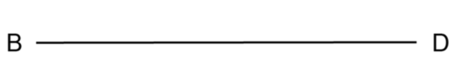### Construct an arc that intersects the line segment $$BD$$.

Place the point of the compass on point $$B$$ and construct a wide arc as shown in the diagram below. The point where the arc intersects the line $$BD$$ is labelled $$C$$.### Construct a second arc that intersects the first arc.

Without changing the width of the compass, place the compass on point $$C$$ and construct a second arc that intersects the first arc. The point where the two arcs intersect is labelled point $$A$$.### Draw line segment $$BA$$.

Use a ruler to draw the line segment $$BA$$ which forms the other arm of the angle.

$$A\hat{B}C = 60^{\circ}$$. We can use a protractor to check the accuracy of the construction.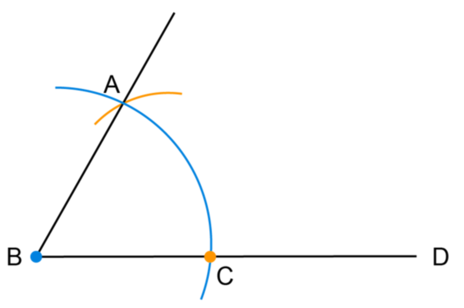In the previous worked example, we constructed a $$60^{\circ}$$ angle. Look at the steps of the construction. Did you notice that $$BC = CA = AB$$? The diagram below shows the final step of the same construction with line segment $$CA$$ drawn. This means that we have constructed an equilateral triangle with all sides equal in length.

$$\triangle ABC$$ is an equilateral triangle with $$BC = CA = AB$$.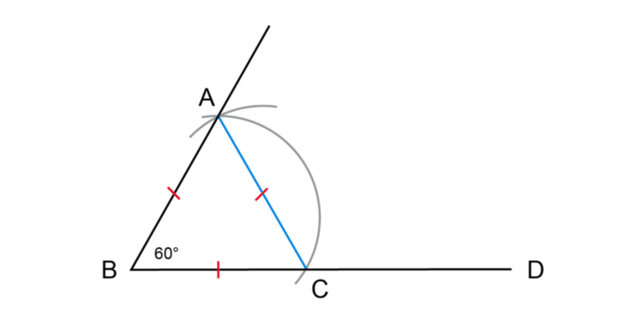To construct a $$30^{\circ}$$ angle using a compass, we first construct a $$60^{\circ}$$ angle and then bisect it to get a $$30^{\circ}$$ angle.

## Worked Example 10.8: Constructing a $$30^{\circ}$$ angle

Use a compass to bisect angle $$A\hat{B}C = 60^{\circ}$$.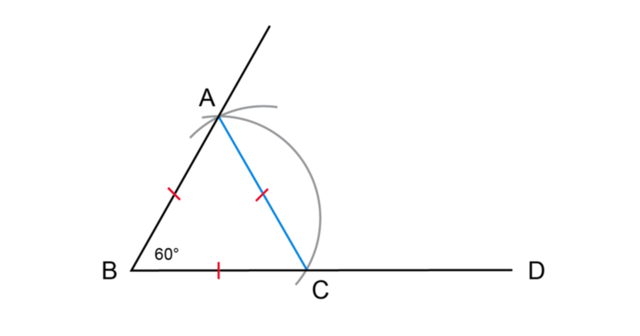### Construct intersecting arcs between the arms of the angle.

Set the width of the compass equal to $$BC$$. Place the compass on point $$C$$ and draw an arc inside the angle. Without changing the compass width, place the compass on point $$A$$ and draw a second arc inside the angle that intersects the first arc (shown as green arcs in the diagram).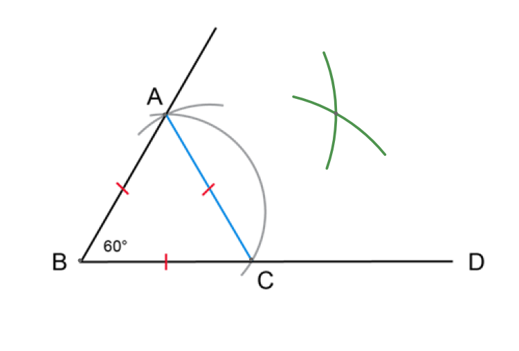### Draw a line from the point of intersection to the vertex of the angle.

Use a ruler to join the vertex (point $$B$$) to the point where the arcs intersect (point $$E$$).

$$BE$$ is the bisector of $$A\hat{B}C$$.

$$C\hat{B}E = E\hat{B}A = 30^{\circ}$$.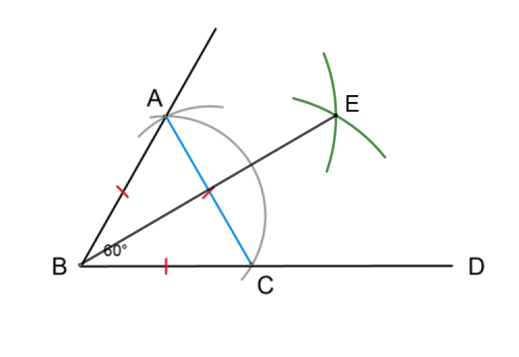### Constructing other angles using $$60^{\circ}$$ and $$30^{\circ}$$

We can construct angles of different sizes by constructing a $$60^{\circ}$$ angle and then:

• bisecting the angle
• adding $$90^{\circ}$$ or subtracting from $$90^{\circ}$$
• adding $$180^{\circ}$$ or subtracting from $$180^{\circ}$$
• or subtracting from $$360^{\circ}$$.

Here are a few examples of different types of angles that can be constructed using $$60^{\circ}$$:

1. Construct $$120^{\circ}$$ using a compass.

First we construct $$60^{\circ}$$ and then subtract the angle from $$180^{\circ}$$: $$180^{\circ} − 60^{\circ} = 120^{\circ}$$

2. Construct $$150^{\circ}$$ using a compass.

First we construct $$60^{\circ}$$ and then bisect the angle to get $$30^{\circ}$$. We can then subtract the angle from $$180^{\circ}$$: $$180^{\circ} − 30^{\circ} = 150^{\circ}$$

3. Construct $$300^{\circ}$$ using a compass.

First we construct $$60^{\circ}$$ and then subtract the angle from $$360^{\circ}$$: $$360^{\circ} − 60^{\circ} = 300^{\circ}$$

4. Construct $$195^{\circ}$$ using a compass.

First we construct $$60^{\circ}$$ and then bisect the angle to get $$30^{\circ}$$. We bisect the angle again to get $$15^{\circ}$$ and then add $$180^{\circ}$$: $$180^{\circ} + 15^{\circ} = 195^{\circ}$$

### Constructing angles using a compass

1. Use a compass to construct $$X\hat{Y}Z = 60^{\circ}$$.
2. Use a compass to construct $$M\hat{N}P = 60^{\circ}$$.
1. Bisect $$M\hat{N}P$$.
2. Use a protractor to check the accuracy of your construction.
3. Construct equilateral triangle $$\triangle JKL$$ with $$JK = 4 \text{ cm}$$.
4. Massah wants to construct the following angles using a compass. Write down each step to show how Massah would use a $$60^{\circ}$$ construction to draw each angle. Express each answer as a calculation. The first question is completed as an example.
1. $$330^{\circ}$$

Step 1: Construct $$60^{\circ}$$ angle.

Step 2: Bisect $$60^{\circ}$$ angle to get $$30^{\circ}$$ angle.

Step 3: Subtract $$30^{\circ}$$ angle from $$360^{\circ}$$ to get $$330^{\circ}$$ angle.

Calculation: $$360^{\circ} − \left( \frac{60^{\circ}}{2} \right) = 330^{\circ}$$

1. $$240^{\circ}$$
2. $$165^{\circ}$$
3. $$\text{7,5}^{\circ}$$
4. $$105^{\circ}$$
5. $$210^{\circ}$$
6. $$345^{\circ}$$
5. Use a compass to construct each angle in Question 4.
6. Use a protractor to check the accuracy of each construction.

### Constructing $$90^{\circ}$$ and $$45^{\circ}$$ angles using a compass

Earlier in this chapter, we constructed a perpendicular line at a given point. We know that perpendicular lines intersect at right angles to each other. So we can use this same method for constructing a $$90^{\circ}$$ angle.

## Worked Example 10.9: Constructing a $$90^{\circ}$$ angle

$$P$$ is a point on the line $$AB$$. Use a compass to construct $$B\hat{P}Q = 90^{\circ}$$.

### Construct two arcs equidistant from point $$P$$.

Place your compass on point $$P$$ and draw an arc on each side of point $$P$$. Make sure to keep the width of the compass exactly the same when drawing both arcs.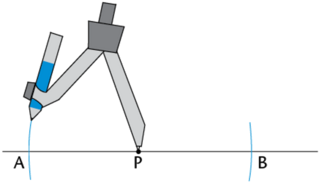### Construct two intersecting arcs above point $$P$$.

Place the compass on each arc and draw an arc above point $$P$$. Label the point of intersection $$Q$$.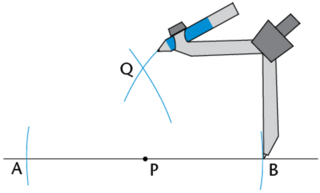### Draw line segment $$PQ$$.

Use your ruler to join $$P$$ and $$Q$$.

$$\therefore B\hat{P}Q = A\hat{P}Q = 90^{\circ}$$.To construct a $$45^{\circ}$$ angle using a compass, we first construct a $$90^{\circ}$$ angle and then bisect it to get a $$45^{\circ}$$ angle.

## Worked Example 10.10: Constructing a $$45^{\circ}$$ angle

Use a compass to bisect angle $$B\hat{P}Q = 90^{\circ}$$.### Construct intersecting arcs between the arms of the angle.

Set the width of the compass equal to $$PB$$. Place the compass on point $$B$$ and draw an arc inside the angle. Without changing the compass width, place the compass on point $$Q$$ and draw a second arc inside the angle that intersects the first arc (shown by the pink arcs in the diagram below). Label point of intersection $$R$$.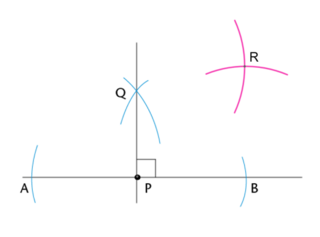### Draw a line from the point of intersection to the vertex of the angle.

Use a ruler to join the vertex (point $$P$$) to the point where the arcs intersect (point $$R$$).

$$PR$$ is the bisector of $$B\hat{P}Q$$.

$$\therefore B\hat{P}R = R\hat{P}Q = 45^{\circ}$$.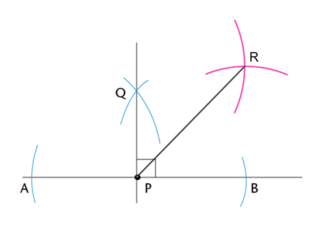### Constructing other angles using $$90^{\circ}$$ and $$45^{\circ}$$

We can construct angles of different sizes by constructing a $$90^{\circ}$$ angle and then:

• bisecting the angle
• adding $$90^{\circ}$$ or subtracting from $$90^{\circ}$$
• adding $$180^{\circ}$$ or subtracting from $$180^{\circ}$$
• or subtracting from $$360^{\circ}$$.

Here are a few examples of different types of angles that can be constructed using $$45^{\circ}$$:

1. Construct $$225^{\circ}$$ using a compass.

First we construct $$90^{\circ}$$ and then bisect the angle to get $$45^{\circ}$$. We can then add $$180^{\circ}$$ to the angle:

\begin{align} 180^{\circ} + \left( \frac{90^{\circ}}{2} \right) &= 225^{\circ} \\ 180^{\circ} + 45^{\circ} &= 225^{\circ} \end{align}
2. Construct $$315^{\circ}$$ using a compass.

First we construct $$90^{\circ}$$ and then bisect the angle to get $$45^{\circ}$$. Then we subtract the angle from $$360^{\circ}$$:

\begin{align} 360^{\circ} − \left( \frac{90^{\circ}}{2} \right) &= 315^{\circ} \\ 360^{\circ} − 45^{\circ} &= 315^{\circ} \end{align}

### Work with constructions

Use a compass to construct $$P\hat{Q}R = 90^{\circ}$$.

1. Bisect $$P\hat{Q}R$$.
2. Use a protractor to check the accuracy of your construction.

Aimee wants to construct the following angles using a compass.

Write down each step to show how Aimee would use a $$90^{\circ}$$ construction to draw each angle.

Express each answer as a calculation.

1. $$135^{\circ}$$
2. $$\text{157,5}^{\circ}$$
3. $$\text{337,5}^{\circ}$$
4. $$75^{\circ}$$

Use a compass to construct each angle in Question 2. Use a protractor to check the accuracy of each construction.

Nompumelelo discovered a different method for constructing a $$90^{\circ}$$ angle using a compass and a ruler. Her final construction is shown below.

1. What was the size of the first angle that Nompumelelo constructed?
2. What was the size of the second angle that Nompumelelo constructed?
3. Using these two angles, how did Nompumelelo obtain a $$90^{\circ}$$ angle?
4. Use the given diagram and your answers to the questions to construct Nompumelelo’s diagram using a compass and a ruler.
5. Use a protractor to check the accuracy of your construction.